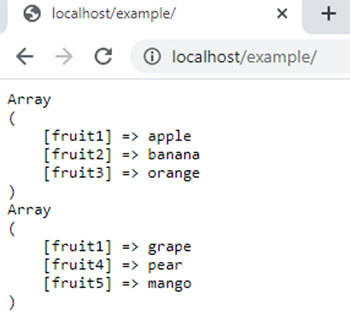array_multisort function in php || php functions

array_multisort() function in PHP

0 1169

array_multisort() function of PHP is used to sort one or more arrays. It is an inbuilt function of PHP. If the array is associative then the keys remains same but in case of numeric keys, this function re-indexed the array from 0.

The return type of thus function is Boolean means it can return either TRUE or FALSE.

Syntax:

array_multisort(\$arr1,\$order_of_sorting,\$type_of_sorting,\$arrr2,\$arr3,....,\$arrN);

Here

1) \$arr1 is a PHP array.

2) \$order_of_sorting parameter used to represents the order of sorting either ascending or descending. It has two values. This parameter is optional.

• SORT_ASC: used to sort array in ascending order. By default it is applied.
• SORT_DESC: used to sort array in descending order.

3) \$type_of_sorting represents the type of sorting. It is also an optional parameter. It has 6 different values.

• SORT_REGULAR: compare items according to Standard ASCII in regular manner.
• SORT_NUMERIC: compare items as numeric values.
• SORT_STRING: compare items as string values.
• SORT_LOCALE_STRING: compare items as string according to locale values.
• SORT_NATURAL: using 'Natural Ordering', it compare items as string.
• SORT_FLAG_CASE: can be combined (bitwise OR) with SORT_STRING or SORT_NATURAL to sort items case-insensitively as string.

4) \$arr2,\$arr3 and \$arrN are PHP arrays which are optional

Example:

<?php
\$arr1 = array("fruit1"=>"apple","fruit2"=>"banana","fruit3"=>"orange");
\$arr2 = array("fruit1"=>"grape","fruit4"=>"pear","fruit5"=>"mango");
array_multisort(\$arr1,\$arr2);
echo "<pre>";
print_r(\$arr1);
print_r(\$arr2);
?>

Output:Share: